Bean语言今天正式开源，代码托管于Github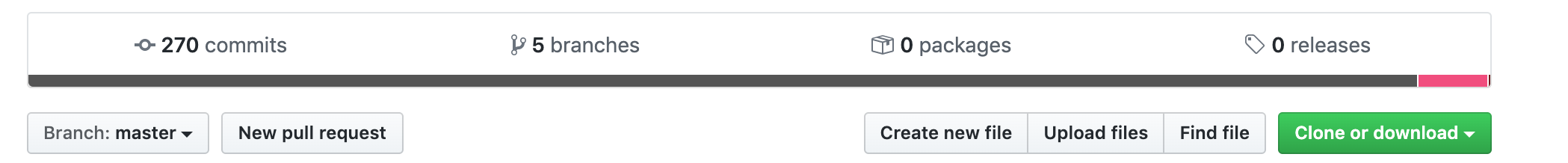Just for fun.

# 技术栈的选型

OK，那剩下来的选择似乎也不多了，C，C++，Go，Rust。最后我选择了C，不选C++的理由很简单，第一眼看上去就难以接受，如此之复杂的语法，很难保证到了最后是自己在写这门语言还是被这门语言给“写”了。业界都喜欢把C跟C++放在一起，但是我觉得他们根本就不是一回事。

# 词法分析器-手写与生成的抉择``````function a()
return 1111
end

print(a()) // => 1111
``````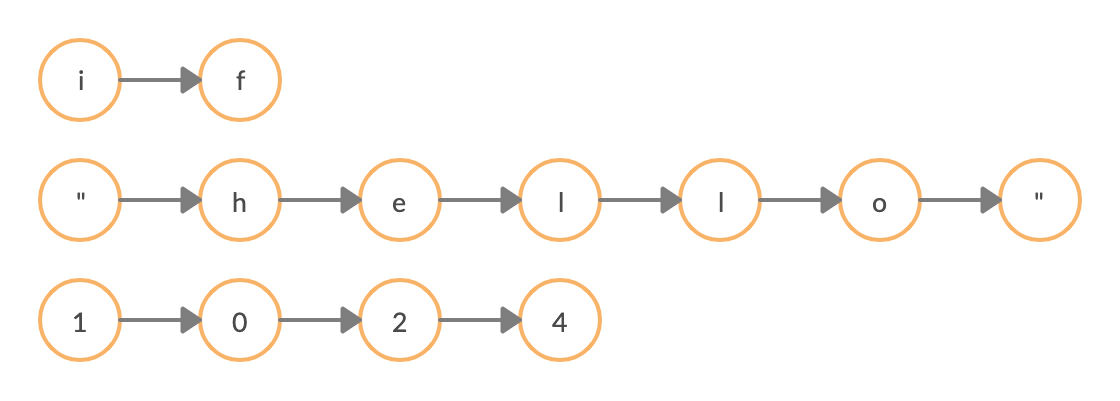# 语法分析器的制作

PS: 我甚至去Github上翻Ruby1.8以前的源代码（在那之前还没采用虚拟机），找找线索，不过Github上的相关记录似乎已经被抹去了。

``````typedef struct expr {
EXPR_TYPE type;

union {
bean_Number nval;  /* for VKFLT */
bu_byte bval;
TString * sval;
Function * fun;

struct {
struct expr * condition;
dynamic_expr * if_body;
dynamic_expr * else_body;
} branch;

struct {
int op; // Store the TokenType
struct expr * left;  /* for   TK_ADD, TK_SUB, TK_MUL, TK_DIV, */
struct expr * right;
int assign; // Need reassign
} infix;

struct {
struct expr * condition;
bool firstcheck;
dynamic_expr * body;
} loop;
}
...
}

``````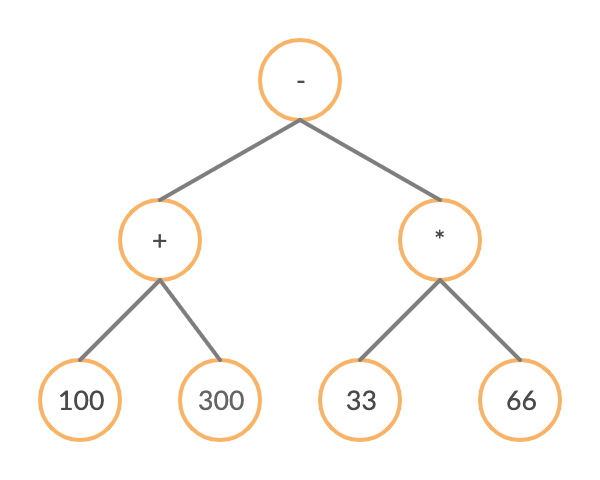## 语法分析器生成器

``````input:
%empty
| input line
;

line:
'\n'
| exp '\n'      { printf ("%.10g\n", \$1); }
;

exp:
NUM
| exp exp '+'   { \$\$ = \$1 + \$2;      }
| exp exp '-'   { \$\$ = \$1 - \$2;      }
| exp exp '*'   { \$\$ = \$1 * \$2;      }
| exp exp '/'   { \$\$ = \$1 / \$2;      }
| exp exp '^'   { \$\$ = pow (\$1, \$2); }  /* Exponentiation */
| exp 'n'       { \$\$ = -\$1;          }  /* Unary minus   */
;
%%
``````

## 解析器-解析抽象语法树

``````eval('100 + 22')
eval('if(a < 10) { a ++ }')
...
``````

``````eval_infix('100 + 200')
eval_if('if(a < 10) { a ++ }')
...
``````

`100`, `22`这种数值的信息本身就是放在抽象语法树里面的，而变量`a`的值则需要从当前运行的上下文中去获取。接下来再看具体一点的例子。假设我有一颗这样的语法树，类型为`INFIX`

``````var num1 {
type: NUMBER,
value: 1
}

var num2 {
type: NUMBER,
value: 100
}

var tree = {
type: INFIX,
left: num1
right: num2,
}
``````

``````function eval(tree) {
switch(tree.type) {
case(INFIX): {
return eval_infix(tree)
}
case(NUMBER): {
return tree.value
}
.....
}
}

function eval_infix(tree) {
switch(tree.op) {
return eval(tree.left) + eval(tree.right)
}
case(TK_SUB): {
return eval(tree.left) - eval(tree.right)
}
.....
}
}
``````

``````> print("Hello World")
Hello World
=> nil
``````

# 基本类型与内置工具库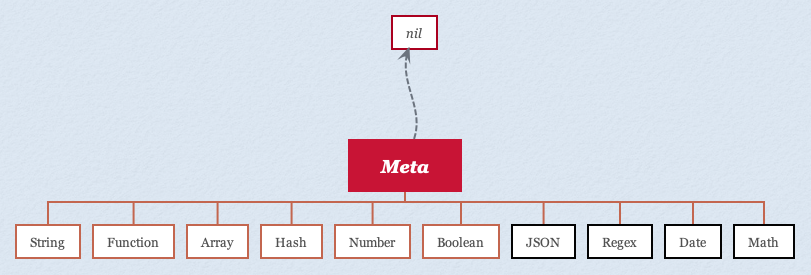``````> typeof nil
=> "nil"
> typeof true
=> "boolean"
> typeof 1
=> "number"
> typeof "string"
=> "string"
> typeof []
=> "array"
> typeof {}
=> "hash"
> typeof fn() {}
=> "function"
``````

# 虚拟机

Matz的原话是这样的

## 虚拟机的实现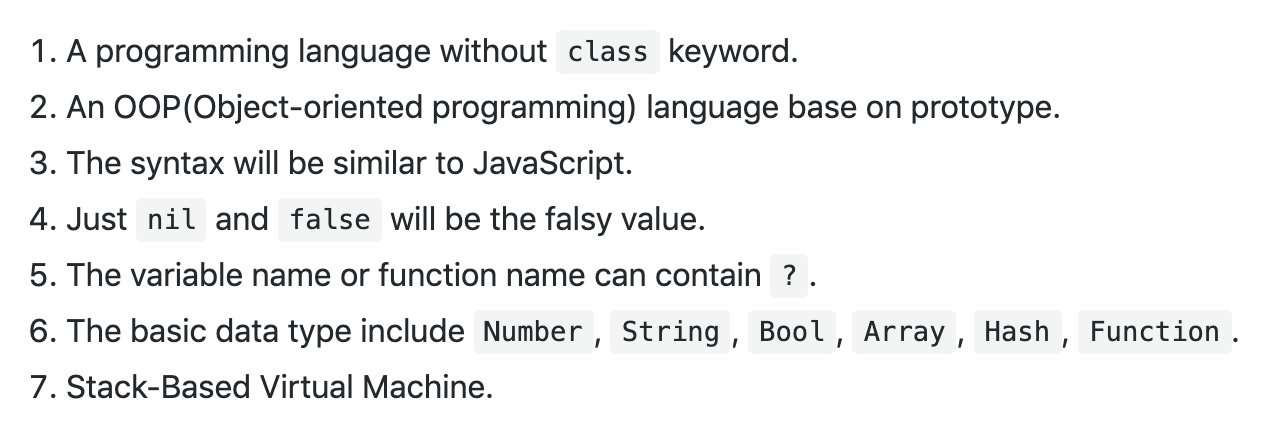``````while(true) {
switch(GET_OP()) {
value1 = POP();
value2 = POP();
PUSH(value1 + value2);
break;
}
case OP_OTHER: {
value1 = DECODE_FROM_STREEM();
value2 = POP();
PUSH(special_handle(value2, value1));
break;
}
}

if (end) break;
}
``````